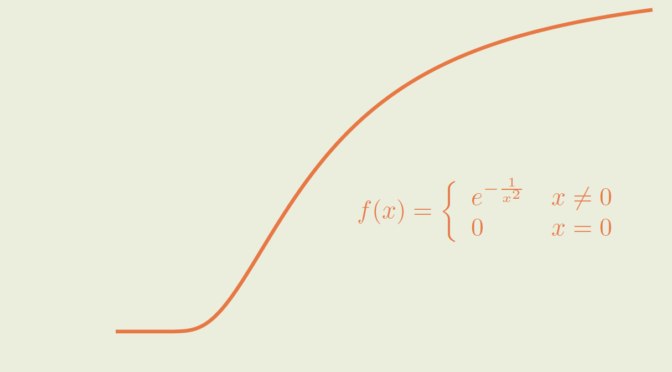# A positive smooth function with all derivatives vanishing at zero

Let’s consider the set $$\mathcal C^\infty(\mathbb R)$$ of real smooth functions, i.e. functions that have derivatives of all orders on $$\mathbb R$$.

Does a positive function $$f \in \mathcal C^\infty(\mathbb R)$$ with all derivatives vanishing at zero exists?

Such a map $$f$$ cannot be expandable in power series around zero, as it would vanish in a neighborhood of zero. However, the answer to our question is positive and we’ll prove that $f(x) = \left\{\begin{array}{lll} e^{-\frac{1}{x^2}} &\text{if} &x \neq 0\\ 0 &\text{if} &x = 0 \end{array}\right.$ provides an example.

$$f$$ is well defined and positive for $$x \neq 0$$. As $$\lim\limits_{x \to 0} -\frac{1}{x^2} = -\infty$$, we get $$\lim\limits_{x \to 0} f(x) = 0$$ proving that $$f$$ is continuous on $$\mathbb R$$. Let’s prove by induction that for $$x \neq 0$$ and $$n \in \mathbb N$$, $$f^{(n)}(x)$$ can be written as $f^{(n)}(x) = \frac{P_n(x)}{x^{3n}}e^{-\frac{1}{x^2}}$ where $$P_n$$ is a polynomial function. The statement is satisfied for $$n = 1$$ as $$f^\prime(x) = \frac{2}{x^3}e^{-\frac{1}{x^2}}$$. Suppose that the statement is true for $$n$$ then $f^{(n+1)}(x)=\left[\frac{P_n^\prime(x)}{x^{3n}} – \frac{3n P_n(x)}{x^{3n+1}}+\frac{2 P_n(x)}{x^{3n+3}}\right] e^{-\frac{1}{x^2}}$ hence the statement is also true for $$n+1$$ by taking $$P_{n+1}(x)= x^3 P_n^\prime(x) – 3n x^2 P_n(x) + 2 P_n(x)$$. Which concludes our induction proof.

Finally, we have to prove that for all $$n \in \mathbb N$$, $$\lim\limits_{x \to 0} f^{(n)}(x) = 0$$. For that, we use the power expansion of the exponential map $$e^x = \sum_{n=0}^\infty \frac{x^n}{n!}$$. For $$x \neq 0$$, we have $\left\vert x \right\vert^{3n} e^{\frac{1}{x^2}} \ge \frac{\vert x \vert^{3n}}{(2n)! \vert x \vert ^{4n}} = \frac{1}{(2n)! \vert x \vert^n}$ Therefore $$\lim\limits_{x \to 0} \left\vert x \right\vert^{3n} e^{\frac{1}{x^2}} = \infty$$ and $$\lim\limits_{x \to 0} f^{(n)}(x) = 0$$ as $$f^{(n)}(x) = \frac{P_n(x)}{x^{3n} e^{\frac{1}{x^2}}}$$ with $$P_n$$ a polynomial function.